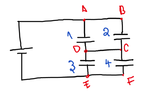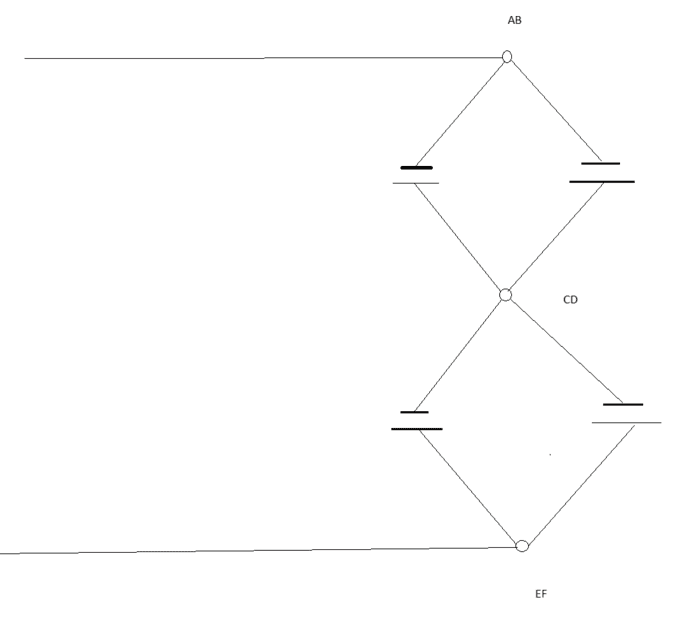# Capacitor Network - Series or Parallel?

Shreya
Homework Statement:
Find the equivalent capacitance.
Relevant Equations:
Series & Parallel Capacitor formulae
My textbook solution states that 1 & 2 are in parallel and so is 3 & 4 and those 2 are in series. That is, (1 P 2) S (3 P 4). My thinking is such: points A & B are of same potential, say V, C & D are of same potential, say x and E & F are are of same potential, say 0. So I can say that 1 and 3 are in series and so is 2 &4. Therefore, I can say (1 S 3) P (2 S 4). I'm sure there is a flaw in my method. Please be kind to point it out.

#### Attachments

•Screenshot from 2022-10-12 19-54-51.png
9.7 KB · Views: 16

Homework Helper
Homework Statement:: Find the equivalent capacitance.
Relevant Equations:: Series & Parallel Capacitor formulae

My textbook solution states that 1 & 2 are in parallel and so is 3 & 4 and those 2 are in series. That is, (1 P 2) S (3 P 4). My thinking is such: points A & B are of same potential, say V, C & D are of same potential, say x and E & F are are of same potential, say 0. So I can say that 1 and 3 are in series and so is 2 &4. Therefore, I can say (1 S 3) P (2 S 4). I'm sure there is a flaw in my method. Please be kind to point it out.
C and D are only guaranteed to be at the same potential because there is a wire connecting them.

If you had (1 S 3) P (2 S 4) then there would be no wire connecting the middle nodes in the two series chains and there would be no guarantee that the two middle nodes would be at the same potential.

If you had such a guarantee (say because the two series chains had capacitors with identical ratios of capacitance) then the two middle nodes would be at the same potential and the circuit would behave identically to the series chain of two parallel pieces (1 P 2) S (3 P 4).

It might be helpful to re-draw the circuit to eliminate the redundant nodes B, D and F (if two nodes are connected by a wire, there is only one node). Then the true nature of the circuit becomes more obvious.

•Shreya
Shreya
That makes sense @jbriggs444 . But I don't see why 1 P 2 would be in series with 3 P 4 though

Gold Member
That makes sense @jbriggs444 . But I don't see why 1 P 2 would be in series with 3 P 4 though
There is no resistance between A and B, C and D, or E and F. You can combine each pair into a single node. The nature of the circuit will become clear when you do this.

•Shreya and jbriggs444
Homework Helper
That makes sense @jbriggs444 . But I don't see why 1 P 2 would be in series with 3 P 4 though•Shreya and erobz
Shreya
Last edited:
Homework Helper
Gold Member
Homework Statement:: Find the equivalent capacitance.
Relevant Equations:: Series & Parallel Capacitor formulae

So I can say that 1 and 3 are in series and so is 2 &4. Therefore, I can say (1 S 3) P (2 S 4). I'm sure there is a flaw in my method. Please be kind to point it out
Two components are in series only if they carry the same current at any time.
That is not the case with 1 and 3 (or 2 and 4).

•Shreya